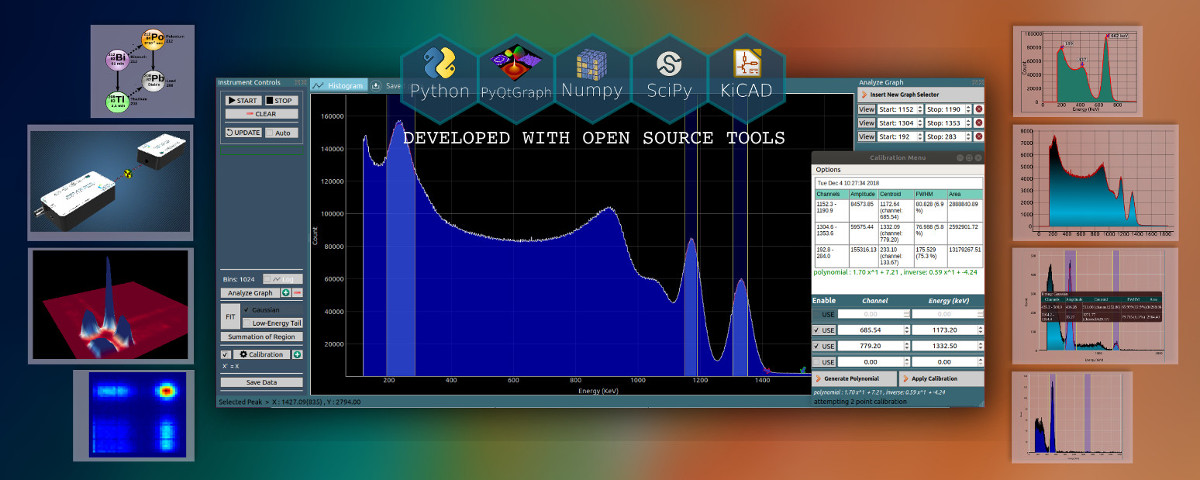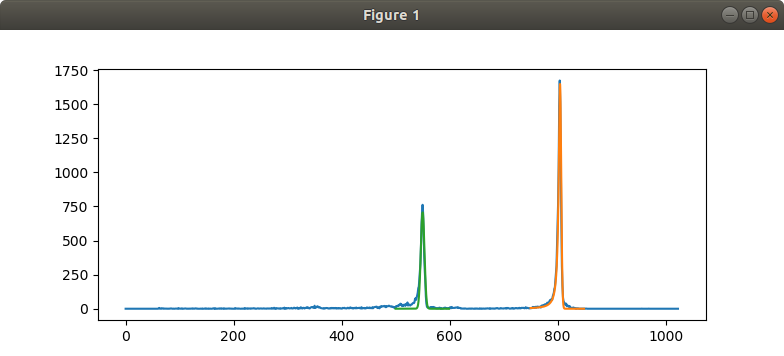# Multi-Channel Analyzer

## 1K and 4K Multi-Channel Analyzers

Multi-Channel Analyzers sort gaussian pulses into channels based on their pulse heights, in order to generate a spectrum. Our compact, USB powered MCAs and accompanying cross-platform, open-source software enables easy visualization and analysis of pulse outputs from various spectrometers.

Tested on Ubuntu 18.04 and Windows 7/10

## Features : 1024 channel MCA

+

3.3V Shaped Input. 3uS rise time.

1024 channels. Fully USB powered unit

16 million counts per channel

8uS processing time per pulse

Fully featured Python software supplied open-source.Dual parameter list mode MCA also available for coincidence measurements

## 4096 channel MCA

+

4V Shaped Input. 3uS rise time.

4096 channels. Fully USB powered unit

2 Bytes per channel.

10uS processing time per pulse including 3uS rise time

Fully featured Python software supplied open-source.#### Software PageOpen-source software, CNSpec, is supplied with the instrument.

#### MCA poster Page### Python Library for flexible operation: Code example

##### Dependencies: Matplotlib(for plotting) , Scipy(For fitting)
``````import time,sys
import numpy as np
from MCALib import connect

dev = connect()

fname = 'DATA/bi212_19mA_10min_31mar18/data_240mins.csv'

# Get the data. Supply an optional name argument in case of multiple files/connected hardware.
x = dev.getHistogram() #name = fname / name='/dev/ttyUSB0'

#Optional-Uncomment next two lines to print the whole array. No decimal Points. Suppress scientific notation
#np.set_printoptions(threshold = np.inf,precision = 0,suppress=True) #
#print (x)

#plot the loaded spectrum
import matplotlib.pyplot as plt
plt.plot(x) #Plot RAW data

FIT = dev.gaussianTailFit([750,850]) #Apply a gaussian+Lorentzian FIT between 700 and 900  channel, and overlay it.
if FIT:
plt.plot(FIT['X'],FIT['Y']) #Plot fitted data
print('Gaussian+low energy tail Fit : ',FIT['centroid'],FIT['fwhm'])

FIT = dev.gaussianFit([500,600]) #Apply a gaussian FIT between 500 and 600 channel.
if FIT:
plt.plot(FIT['X'],FIT['Y']) #Plot fitted data
print('Gaussian Fit : ',FIT['centroid'],FIT['fwhm'])

plt.show()

``````

## Results from offline data analysis

The results of the above code on offline analysis of 212-Bismuth spectrum are shown

Gaussian fitting was carried out on the first peak(Green) and overlaid.

Gaussian+Low energy tail(Lorentzian) was carried out on the second peak (Orange).The Python library is used to encourage students to understand the acquired data, and interpret and analyze it manually instead of relying on the graphical interface. However, the GUI is far more efficient, and feature rich.

#### Terminal output [Centroid, FWHM]

``````Gaussian+low energy tail Fit :  804.5779218431603 5.52159634760166
Gaussian Fit :  550.0967122469116 7.927848067452287
``````
• e-mail csparkresearch@gmail.com for enquiries